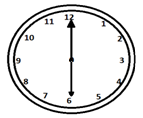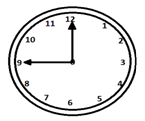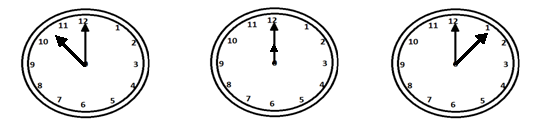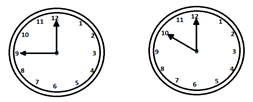# Reasoning - Clock

The hands of a clock can have a maximum angle of 180o between them. When this happens, both the hands represent a straight line.The hands of a clock make right angles twice every hour when they are 15 minutes away from each other.The two hands of a clock coincide once in every hour.A clock is very essential for every human being to schedule their daily tasks. The clock theory is related to everyday life.

A clock is an instrument that displays time by dividing it into hours, minutes, and seconds.

### Dial

It has a circular dial numbered from 1 to 12 that indicates the hours. The circumference of a dial is further divided into 60 equal spaces, that are called the minutes’ spaces.

1 hour = 60 minutes

1 minute = 60 seconds and

1 hour = 3600 seconds

### Tricks

• Between n and (n+1) Oclock, the two hands of a clock will coincide at $\left ( \frac{60n}{11} \right )$ min past n.

• Between n and (n+1) Oclock, the two hands of a clock will mutually make a right angle at $\left ( 5n \pm 15 \right )\times\frac{12}{11}$ min past n.

• Between n and (n+1) Oclock, the hands of a clock will be in a straight line (without being together) at

• $\left ( 5n - 30 \right )\times\frac{12}{11}$ min past n,(when n >6)

• $\left ( 5n + 30 \right )\times\frac{12}{11}$ min past n,(when n <6)

• Between n and (n+1) Oclock, the hands of a clock are $x$ min apart at $\left ( 5n \pm x \right )\times\frac{12}{11}$ min past n. ‘+’ sign indicates that the minute hand is ahead and ‘–‘ sign indicates that the hour hand is ahead.

• If the minute hand of a clock overtakes the hour hand at an interval of $x$ min of the correct time, then the clock loses or gains by $\left ( \frac{720}{11} - x \right )\left ( \frac{60 \times 24}{x} \right )$ min.

1 − At what time between 7.15 and 8.15, the hands of a clock will coincide each other?

Options

A - $39\frac{5}{11}$

B - $39\frac{4}{11}$

C - $38\frac{5}{11}$

D - $39\frac{4}{11}$

Explanation

$\frac{60 \times n}{11} = \frac{60}{11} \times \frac{29}{4} = 39\frac{5}{11}$

Where $n = 7.15 = 7\frac{15}{60} = \frac{29}{4}$

2 − In what particular time, between 9 and 8 O’clock, both the hands will be at a right angle to each other?

Options

A - $65\frac{4}{11}$ and $32\frac{7}{11}$ min past 9

B - $65\frac{2}{11}$ and $32\frac{2}{11}$ min past 9

C - $65\frac{3}{11}$ and $32\frac{3}{11}$ min past 9

D - $65\frac{1}{11}$ and $32\frac{1}{11}$ min past 9Explanation

$\left ( 5n \pm 15 \right ) \times\frac{12}{11} = \left ( 5\times9\pm 15 \right )\times\frac{12}{11}$

$= 65\frac{4}{11}$ and $32\frac{7}{11}$ min past 9

3 − In between 12 and 1 O`clock, when will both the hands of a clock form a straight line?

Options

A - $\frac{360}{11}$ min. past 12

B - $\frac{355}{11}$ min. past 12

C - $\frac{340}{11}$ min. past 12

D - $\frac{345}{11}$ min. past 12

Explanation

$\left ( 5n-30 \right )\times\frac{12}{11}$ min past n

$\left ( 6-30 \right )\times\frac{12}{11}$ min. past 12

$= \frac{345}{11}$ min. past 12 (since n = 12)

4 − In how much time, hands of a clock will be in the 30 min. space apart when they are in between 12 and 1 p.m. on Monday?

Options

A - $\frac{1080}{11}$ and $\frac{300}{11}$ min past 12

B - $\frac{1080}{12}$ and $\frac{360}{11}$ min past 12

C - $\frac{1080}{11}$ and $\frac{360}{11}$ min past 12

D - $\frac{1080}{12}$ and $\frac{300}{12}$ min past 12

Explanation

$\left ( 5n\pm x \right )\times\frac{12}{11}$

$= \left ( 5\times12\pm 30 \right )\times\frac{12}{11}$

$= \frac{90\times12}{11}$ and $\frac{30\times12}{11}$ min past 12 = $\frac{1080}{11}$ and $\frac{360}{11}$ min past 12

5 − The minute hand of a clock overtakes the hour hand at interval of 61 min. then the clock loses or gain by how much time?

Options

A - $104\frac{4}{671}$ min.

B - $105\frac{1}{671}$ min.

C - $104\frac{3}{671}$ min.

D - $105\frac{4}{671}$ min.

Explanation

$\left ( \frac{720}{11}-61 \right )\times\left ( \frac{60\times24}{61} \right )$

$= 105\frac{1}{671}$ min.

reasoning_clock.htm